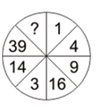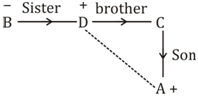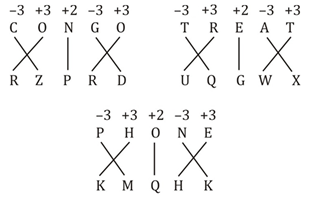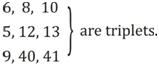# SSC Reasoning Ability Practice Questions (Day-71)

Dear Aspirants, The SSC Exams are going on at national level. Millions of candidates have eagerly prepared for the ongoing SSC exams such as CGL, CHSL, MTS, CPO, JE etc. SSC exams are usually conducted by the Staff Selection Commission to recruit the eligible candidates for various Central government departments. Mostly the selection process for all the SSC exams consists of the single or double phase of written examination based on the examination. Due to that, the written exam consisted of a certain syllabus and exam pattern. By following those regulations, the written exam has been conducted through an online mode. Based on the latest updated exam pattern and syllabus, here we have drafted the Reasoning Ability questions on a daily basis. So aspirants start your practice session with us and mould your skill in a perfect shape.

Start Quiz

1) If ‘P 3 Q’ means ‘Q is daughter of P’, ‘P 5 Q’ means ‘Q is son of P’, ‘P 7 Q’ means ‘P is sister Q’, ‘P 9 Q’ means ‘P is brother of Q’. Which of the followingexpression indicates A is nephew of D?

B 9 D 5 C 5 A

B 7 D 7 C 3 A

B 7 D 9 C 5 A

B 7 D 9 C 3 A

Direction (2-3): In the following question, select the word which cannot be formed using the letters of the given word.

2) ENCOURAGING

(a) GRAIN

(b) RAGING

(c) GAUGE

(d) ENCOURAGE

3) LANGUAGE

(a) SLANG

(b) GAUGE

(c) GLANE

(d) GANG

4) In a certain code language, “RESTED” is written as “SDTSFC” and “POINTS” is written as “QNJMUR”. How is “BANNED” written in that codelanguage?

(a)CZOMFC

(b) ABMODE

(c) CZOODE

(d) ABMMFC

5) In a certain code language, “CONGO” is written as “RZPRD” and “TREAT” is written as “UQGWX”. How is “PHONE” written in that code language?

(a) JNQIJ

(b) KMQHK

(c) MKQKH

(d) LLPIL

6) If “@” denotes “added to”, “#” denotes “multiplied by”, “®” denotes “divided by” and “%” denotes “subtracted from”, then which of the following equation is true?

(a) 8 @ 8  ® 8 # 8 % 8 = 9

(b) 42 % 26 ® 13 # 2 @ 8  = 46

(c) 19 % 84 ® 4 @ 3 # 4 = 12

(d) 31 % 4 ® 2 # 19 @ 3 = 4

7) If “A” denotes “added to”, “B” denotes “subtracted from”, “C” denotes “multiplied by” and “D” denotes “divided by”, then which of the followingequation is true?

(a) 68 D 4 A 6 B 3 C 8 = 0

(b) 13 B 6 D 3 C 2 A 5 = 12

(c) 72 D 18 C 14 B 68 A 10 = – 4

(d) 12 A 6 B 3 C 4 D 3 = 14

8) If 6 # 8 = 10 and 5 # 12 = 13, then 9 # 40 = ?

(a) 47

(b) 63

(c) 41

(d) 53

9) If 7 (110) 4 and 19 (930) 12, then what is the value of ‘A’ in 16 (A) 9?

(a) 580

(b) 600

(c) 640

(d) 700

Direction (10): In the following question, select the number which can be placed at the sign of question mark (?) from the given alternatives.

10)(a) 84

(b) 91

(c) 83

(d) 95

B 7 D 9 C 5 AA is the nephew of D.

‘ENCOURAGE’; there is no double ‘E’ in the given word.

‘SLANG’ ; There is no ‘S’ in the given word.

+1, –1 series42% 26 ® 13 # 2 @ 8 = 46

⇒ 42 – 26 ÷ 13 × 2 + 8 = 46

⇒ 42 – 4 + 8 = 46

⇒ 50 – 4 = 46

⇒ 46 = 46

12 A 6 B 3 C 4 D 3 = 14

⇒ 12 + 6 – 3 × 4  3 = 14

⇒ 12 + 6 – 4 = 14

⇒ 18 – 4 = 14

⇒ 14 = 14(7 + 4) × (7 + 4 – 1) = 110

(19 + 12) × (19 + 12 – 1) = 930

(16 + 9) × (16 + 9 – 1) = 600

1 × 2 + 1 = 3

4 × 3 + 2 = 14

9 × 4 + 3 = 39

16 × 5 + 4 = 84

 Check Here to View SSC CGL / CHSL 2021 Reasoning Ability Questions Day – 70 Day – 69 Day – 68